## Introduction

Induced nonlinear scattering is fundamental to weak turbulence and impacts plasma evolution when wave amplitudes are above a nonlinear threshold. It is particularly important to the dynamics of space plasmas. This kinetic nonlinear wave-particle interaction has been shown to lead to a magnetospheric cavity1 that can amplify whistler waves during active periods2 and regulate the trapped electron population in the radiation belts. It can also influence the evolution of solar wind turbulence3 and explain the longevity of electron beams in the solar wind4.

Generalization of the electrostatic theory of induced nonlinear scattering of lower hybrid waves5 to include the electromagnetic effects6 showed that electrostatic waves in the intermediate frequency range, Ωi < ω < Ωei,e are the ion and electron gyrofrequencies), with a large wave normal angle may be scattered by a resonant nonlinear interaction with thermal electrons into electromagnetic waves with smaller wave normal angles6,7. This Report describes the first laboratory experiment that demonstrates the nonlinear conversion process.

This conversion process forms the theoretical basis1 for producing electromagnetic energy in the ionosphere by seeding weak turbulence of lower hybrid waves and scattering them into whistlers. The whistlers with large group velocity can propagate out of the ionosphere and transport energy over an extended volume to affect the plasma dynamics at remote locations. This suggests the possibility of active radiation belt remediation1,7,8. Since nonlinear scattering is key to a number of important plasma processes, the primary objective of our experiment is to isolate and validate this mechanism in a controlled and repeatable laboratory experiment.

The observed effect that will be discussed in this Report is determined to be nonlinear induced scattering by particles on the basis of three key features. (1) Given sufficient amplitude of a pump wave with frequency fpump and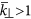, a new spectral feature, the scattered wave, is observed at a downshifted frequency fpump − Δf and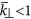, while no corresponding low frequency wave is seen at the frequency Δf, where the normalized perpendicular wave vector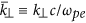, ωpe is the electron plasma frequency and c is the speed of light. (2) The amplitude of the scattered wave exhibits a quadratic dependence on the pump wave amplitude above a threshold, below which no scattered wave is observed. (3) The beat wave that would be present between the pump and scattered waves is in Landau resonance with the background thermal electrons 2πΔf/(vteΔkz) ~ 1, where vte is the electron thermal speed and Δkz is the absolute value of the difference between the parallel wave vectors of the pump and scattered waves. Furthermore, the perpendicular wavelength of the scattered waves is an order of magnitude larger than that of the pump wave and longer than the electron skin depth.

The experiments were conducted in the Space Physics Simulation Chamber (SPSC) at the Naval Research Laboratory (NRL)9,10 in the main chamber section, which is a 5-m long, 2-m diameter cylindrical vacuum chamber with a large area hot filament plasma source. The operational parameters for the steady-state argon plasma are plasma density n = 3 × 1010 cm−3, ion and electron temperatures Ti ≈ 0.05 eV and Te = 1.3 eV, respectively and uniform axial magnetic field B = 5 mT. The electron-neutral collision frequency is νen ≈ 106 s−1 for a neutral density of nn ~ 1013 cm−3. These parameters correspond to scaled conditions of low-β (~6 × 10−4) and dense (ωpee ~ 11) plasma. The effective plasma column diameter and length over which the plasma is observed to be uniform are 1 m and 5 m, respectively.

The plasma measurements were made with probes that are scanned in a two-dimensional plane, 2 m along the axis of the chamber and 60 cm in one direction perpendicular to the background field. A guarded Langmuir probe was used to measure density and electron temperature. Three-axis magnetic field probes were used to measure the wave fields. The pump wave was launched using a 1.2-m-long linear antenna aligned axially and positioned at the radial edge of the plasma column. The antenna consists of four 30 cm × 30 cm panels that can be independently phased. For all of the experiments presented, the first and third panels and the second and fourth panels were electrically connected, while the two pairs of panels were driven 180° out of phase. The scattered waves are observed for a range of pump frequencies. See Fig. 1 for a schematic of the experimental setup.

Figure 2 depicts typical spectra for an experimental run where the pump wave amplitude is above (solid black) and just below (dashed red) the nonlinear scattering threshold. They were measured approximately 15 cm from the antenna. The magnitude of the measured magnetic field is plotted as a function of frequency. The pump wave at fpump = 10 MHz is truncated in the large amplitude case with peak at 159 nT (δB/B ≈ 3 × 10−5). The spectral feature peaking at f ~ fpump − Δff = 40 kHz) is the induced scattered signal. The pump wave amplitude decreases by about an order of magnitude outside the resonance cone, but it is measureable over the plasma column. The peak amplitude of the scattered wave occurs far from the antenna near the center of the plasma column, not where the pump wave amplitude peaks. The relatively broadband continuous nature of the scattered signal is due to the ease of satisfying the Landau resonance condition of the beat waves. There is no corresponding low frequency spectral feature at f ~ Δf indicating that this is not a three-wave process.

If the sideband at 9.96 MHz were due to a three-wave decay process, we can estimate the expected magnitude of the wave magnetic field of the low frequency (40 kHz) wave, by using the fact that the plasmon density N = W/ω of the two daughter waves in parametric three-wave decay should be equal. We use the cold plasma dispersion relation to estimate the wave energy density W for the sideband and low frequency wave. We estimated the expected magnitude of the low frequency wave to be 3 nT, which would be measureable above the noise floor of approximately 0.5 nT.

The plot in Fig. 3 shows the normalized peak scattered wave amplitude as a function of normalized pump wave amplitude for RF voltages from 0.07–1.0 V applied to the antenna. The plot demonstrates the nonlinear threshold for the scattering with a value of δB/B = 5 × 10−6. These observations confirm the theoretical predictions1 that a large amplitude is not required to initiate nonlinear induced scattering as long as the scattering rate exceeds the linear damping rate. The plot also indicates a nonlinear dependence on the normalized pump wave amplitude and shows the corresponding parabolic best fit (dashed line). Applying a linear fit results in a mean squared error that is an order of magnitude larger than that from the parabolic fit. Since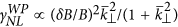7, this confirms that the scattered waves are due to a nonlinear wave-particle mechanism, as a linear dependence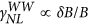would follow from three-wave parametric decay11.

The cross-correlation between a stationary reference probe and a probe moved within the experimental volume is used to map the spatial variation of the magnitude and phase of the launched and scattered waves. Spatial Fourier transforms of this data yield the k-spectrum for these waves. A summary of these results is shown in Fig. 4, where the normalized power spectrum is plotted as a function of k (top pane) and k|| (bottom pane) both normalized to the electron skin depth cpe. The dominant wave mode for the pump wave (dashed red line) exhibited a k|| = −7 ± 2 m−1 and k = 82 ± 14 m1, results in a wave normal angle θ ~ 85°. The wave normal angle is defined as the angle between k and the magnetic field. The spatial Fourier transform indicates 1–2 dominant modes, which allows us to fit to the phase data to achieve better wave vector resolution than the total travel would initially indicate. The perpendicular wave vector normalized to the electron skin depth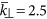demonstrates that the launched wave is predominantly electrostatic.

Cross-correlation measurements for the scattered waves were obtained by averaging an increased number of shots: 100,000 shots were used to remove the random nature present in the waves. Figure 4 shows the large change in perpendicular wave vector that is observed between the pump and scattered waves. The pump wave at 10 MHz with k = 82 m−1 is scattered to waves with k = 10 m−1. The dominant scattered wave was observed to have kz = ±6 m−1, resulting in a wave normal angle θ ~ 59°. The intermediate frequency cold plasma dispersion relation for the experimental parameters is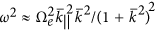. The shaded region in Fig. 4 indicates where the waves are predominantly electrostatic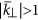with a dispersion of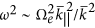(i.e. electrostatic whistlers); the pump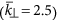scatters into electromagnetic waves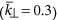with dispersion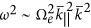(i.e. oblique electromagnetic whistlers).